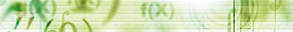Journal metrics
Acceptance rate14%
Submission to final decision82 days
Acceptance to publication37 days
CiteScore0.300
Impact Factor-

### Investigation of a Mild Solution to Coupled Systems of Impulsive Hybrid Fractional Differential Equations

####Journal profile

International Journal of Differential Equations publishes research on differential equations, and related integral equations, from all scientists who use differential equations as tools within their own discipline.

####Editor spotlight

International Journal of Differential Equations maintains an Editorial Board of practicing researchers from around the world, to ensure manuscripts are handled by editors who are experts in the field of study.

####Special Issues

Do you think there is an emerging area of research that really needs to be highlighted? Or an existing research area that has been overlooked or would benefit from deeper investigation? Raise the profile of a research area by leading a Special Issue.

## Latest Articles

More articlesResearch Article

### Solving Linear Second-Order Singularly Perturbed Differential Difference Equations via Initial Value Method

In this paper, an initial value method for solving a class of linear second-order singularly perturbed differential difference equation containing mixed shifts is proposed. In doing so, first, the given problem is modified in to an equivalent singularly perturbed problem by approximating the term containing the delay and advance parameters using Taylor series expansion. From the modified problem, two explicit initial value problems which are independent of the perturbation parameter are produced; namely, the reduced problem and the boundary layer correction problem. These problems are then solved analytically and/or numerically, and those solutions are combined to give an approximate solution to the original problem. An error estimate for this method is derived using maximum norm. Several test problems are considered to illustrate the theoretical results. It is observed that the present method approximates the exact solution very well.

Research Article

### On Some Sufficiency-Type Global Stability Results for Time-Varying Dynamic Systems with State-Dependent Parameterizations

This paper formulates sufficiency-type global stability and asymptotic stability results for, in general, nonlinear time-varying dynamic systems with state-trajectory solution-dependent parameterizations. The stability proofs are based on obtaining sufficiency-type conditions which guarantee that either the norms of the solution trajectory or alternative interval-type integrals of the matrix of dynamics of the higher-order than linear terms do not grow faster than their available supremum on the preceding time intervals. Some extensions are also given based on the use of a truncated Taylor series expansion of chosen truncation order with multiargument integral remainder for the dynamics of the differential system.

Research Article

### Advanced Analytical Treatment of Fractional Logistic Equations Based on Residual Error Functions

In this article, an analytical reliable treatment based on the concept of residual error functions is employed to address the series solution of the differential logistic system in the fractional sense. The proposed technique is a combination of the generalized Taylor series and minimizing the residual error function. The solution methodology depends on the generation of a fractional expansion in an effective convergence formula, as well as on the optimization of truncated errors, , through the use of repeated Caputo derivatives without any restrictive assumptions of system nature. To achieve this, some logistic patterns are tested to demonstrate the reliability and applicability of the suggested approach. Numerical comparison depicts that the proposed technique has high accuracy and less computational effect and is more efficient.

Research Article

### Variational and Numerical Analysis for Frictional Contact Problem with Normal Compliance in Thermo-Electro-Viscoelasticity

In this paper, we consider a mathematical model of a contact problem in thermo-electro-viscoelasticity with the normal compliance conditions and Tresca’s friction law. We present a variational formulation of the problem, and we prove the existence and uniqueness of the weak solution. We also study the numerical approach using spatially semidiscrete and fully discrete finite element schemes with Euler’s backward scheme. Finally, we derive error estimates on the approximate solutions.

Research Article

### Analysis of a Stochastic SIR Model with Vaccination and Nonlinear Incidence Rate

We expand an SIR epidemic model with vertical and nonlinear incidence rates from a deterministic frame to a stochastic one. The existence of a positive global analytical solution of the proposed stochastic model is shown, and conditions for the extinction and persistence of the disease are established. The presented results are demonstrated by numerical simulations.

Research Article

### The Existence and Uniqueness of Intuitionistic Fuzzy Solutions for Intuitionistic Fuzzy Partial Functional Differential Equations

In this paper, we consider intuitionistic fuzzy partial functional differential equations with local and nonlocal initial conditions using the Banach fixed point theorem. A new complete intuitionistic fuzzy metric space is proposed to investigate the existence and uniqueness of intuitionistic fuzzy solutions for these problems. We use the level-set representation of intuitionistic fuzzy functions and define the solution to an intuitionistic fuzzy partial functional differential equation problem through a corresponding parametric problem and further develop theoretical results on the existence and uniqueness of the solution. An example is presented to illustrate the results with some numerical simulation for -cuts of the intuitionistic fuzzy solutions: we give the representation of the surface of intuitionistic fuzzy solutions.Journal metrics
Acceptance rate14%
Submission to final decision82 days
Acceptance to publication37 days
CiteScore0.300
Impact Factor-

You are browsing a BETA version of Hindawi.com. Click here to switch back to the original design.